Next: Detection of Previously Unknown Up: Point Source Sensitivity Previous: Off-axis

### Existence of a Source - Poissonian Statistics Case

The typical HRI background in on-axis detection cells (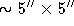) is of order unity or lower for most HRI observations (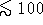ks), and therefore in the range where Poisson statistics are appropriate for source-detection analysis.   For example, the number of background counts expected in a detection cell for a 20 ks observation is 0.55. A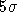detection of a source using Poissonian statistics would then require 7 counts, for a count rate of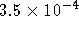counts s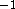in the detection cell, a total count rate of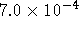counts s. The count rate of the source, however, is known to less than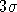. In lieu of an analytic function (of which none exists), Fig. 5.23a displays a contour plot of limiting (total) count rate for a source detection on a grid of significance (sigma) and exposure. The plot was generated for the on-axis detection cell and assumes the nominal background rate. Figure 5.23b shows the same information for a region of interest 10' off axis where the detection cell is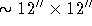and the background count rate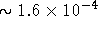counts s. Off axis, the same considerations of vignetting correction and larger detection cells as for the Gaussian case must be taken. The conversion between count rate and flux is the same as above.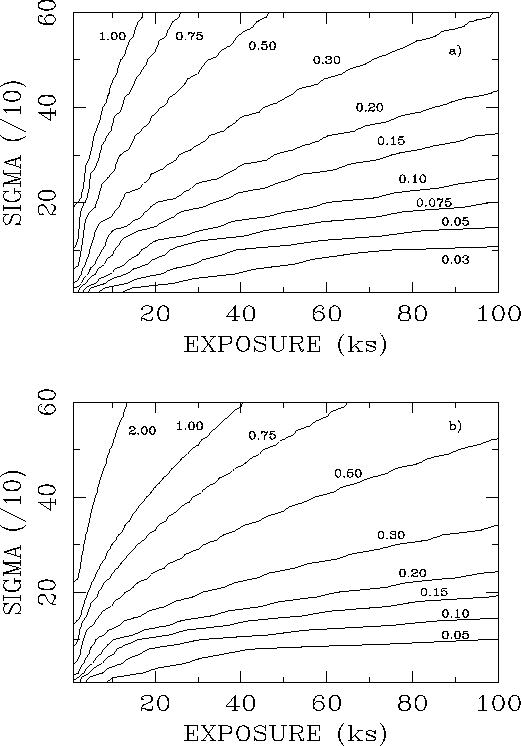Figure 5.23: The relation between source count rate, statistical significance, and exposure for an HRI observation using Poisson statistics is shown. The contour plots show the source count rate in units ofcounts sversus exposure in ks and sigma (a value for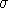of 50 refers to 5). Plot a) is for the on-axis case, plot b) is for 10' off axis.

If you have problems/suggestions please send mail to rosat_svc@mpe-garching.mpg.de